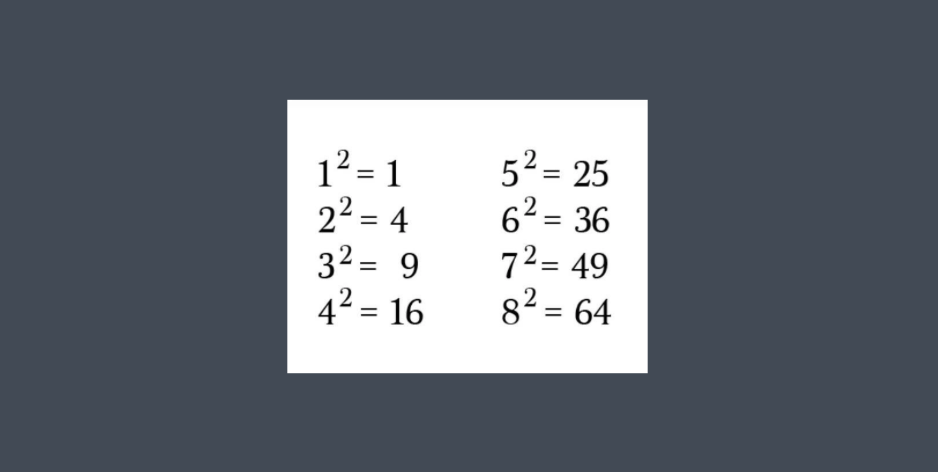# Check if Given Number is a Perfect Square in JavaA perfect square is a number that is made by squaring a whole number.

For example:

16 is a perfect square because 42 = 16.
25 is also a perfect square because 52 = 25.

And it goes on and on … .

So now let’s determine in Java if a given number is perfect square or not.

``````public class checkPerfectSquare(){

public static void main(String args[]){
System.out.println(isPerfectSquare(16));
}
public static String isPerfectSquare(int n) {

double sqrt=Math.sqrt(n);
if ((sqrt - Math.floor(sqrt)) == 0){
return "Integer "+ n+ " is a Perfect Square";
}else{
return "Integer "+ n+ " is NOT a Perfect Square";
}

}
}

//16 is a Perfect Square``````